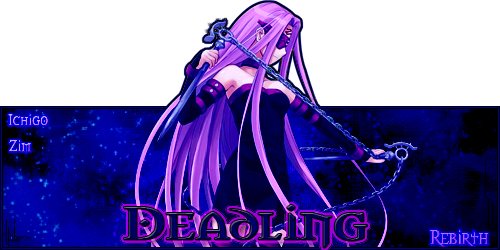## Pets Growth

Where all the good information is!

Moderator: Mods

### Pets Growth

Your pets growth is easy to determine. A pets growth is a very simple math problem basically. Just look at this way. I'll use my own str/str pet for this.
Currently my pet's str is 183, and is lvl 48. I'm going to treat this as if it's starting stat was 2.
You take it's current stat, which would be 183, and minus the starting stat of 2. This leaves it with an end result of 181. (183-2=181)
You then take the pets level, which is 48, and minus 1, making 47. The reason for this is because it's first lvl and stat was never technichally grown, so shouldnt be apart of the final equation.
So in the end, you're left with 181 for it's stat, and 47 for it's lvl. You then divide the stat with the lvl. So in this case, 181/47=3.8xxxxxxx(everything past the first number after the point is not needed.)
So as a result, my pet's growth is 3.8. Not the best growth, but it is what it is. Also if your pet grows in 2 different stats, then it's the same principle. Except that you will have to minus it's starting stats for both stats it grows in, and then add those 2 stats together.

So a rundown of this.
(current stat) -minus- (starting stat) = X
(current lvl) -minus- (starting lvl) = Y
X -divided by- Y = your pets growth.

----------------------------------------------------------------------
Figured this should be posted here, lol.BeastMaster - Zim 7x : Traders: GIR, Dib : BladeMaster - Ichigo 7x